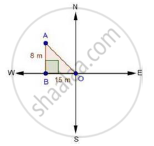Share

# A Man Goes 15 Metres Due West and Then 8 Metres Due North. How Far is He from the Starting Point? - CBSE Class 10 - Mathematics

ConceptApplication of Pythagoras Theorem in Acute Angle and Obtuse Angle

#### Question

A man goes 15 metres due west and then 8 metres due north. How far is he from the starting point?

#### SolutionLet the starting point of the man be O and final point be A.

∴ In ΔABO, by Pythagoras theorem AO2 = AB2 + BO2

⇒ AO2 = 82 + 152

⇒ AO2 = 64 + 225 = 289

⇒ AO = sqrt289 = 17m

∴ He is 17m far from the starting point.

Is there an error in this question or solution?

#### Video TutorialsVIEW ALL 

Solution A Man Goes 15 Metres Due West and Then 8 Metres Due North. How Far is He from the Starting Point? Concept: Application of Pythagoras Theorem in Acute Angle and Obtuse Angle.
S